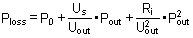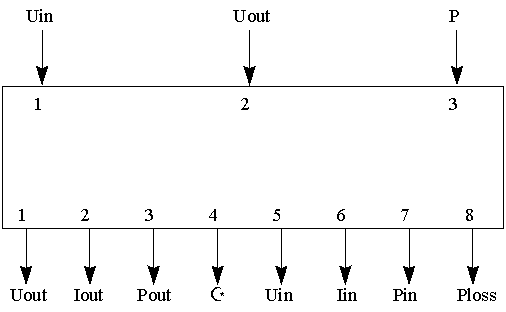TYPE 75: ELECTRIC CONVERTER

General Description

This subroutine models a DC/AC inverter and a DC/DC converter after /1/.

Nomenclature

mode - 1 = Connected to the electric power net 2 = Connected to a load
Z - Power efficiency of the converter or inverter
Pn - Characteristic output power for one module
P - Power ; mode = 1 : Input power known, mode = 2 : Output power known
P0pn - Idling constant for a converter or inverter module (P0pn = P0/Pn, P0 [W] power loss when there is a voltage over the inverter or converter)
Us - Set voltage for a module
Ripn - Inner resistance constant for a Module of converter or inverter (Ripn = Ri*Pn, Ri [Ohm] Inner resistance for a module)
mp - Number of parallel connected modules
Uin - Voltage in to the converter or inverter
Uout - Voltage out of the converter or inverter
Iout - Current out of the converter or inverter
Pout - Power out of the converter or inverter
Iin - Current in to the converter or inverter
Pin - Power in to the converter or inverter
Ploss - The power loss in the converter or inverter

Mathematical Description

The power loss for both modes is calculated with this equation:The inverter/converter can have either output or input power as input for the calculations (output if the system is connected to a load or input if the system is connected to the electric power net).

The converter/inverter is disconnected when the input voltage, output voltage or power is zero.

TRNSYS Component Configuration

PARAMETER NO. DESCRIPTION

1 mode - 1 = Connected to the electric power net 2 = Connected to a load
2 Pn - Characteristic output power for one module
3 P0pn - Idling constant for a converter or inverter module (P0pn = P0/Pn, P0 [W] power loss when there is a voltage over the inverter or converter)
4 Us - Set voltage for a module
5 Ripn - Inner resistance constant for a Module of converter or inverter (Ripn = Ri*pn, Ri [Ohm] Inner resistance for a module)
6 mp - Number of parallel connected modules

INPUT NO. DESCRIPTION

1 Uin - Voltage in to the converter or inverter
2 Uout - Voltage out of the converter or inverter
3 P - Power ; mode = 1 : Input power known, mode = 2 : Output power known

OUTPUT NO. DESCRIPTION

1 Uout - Voltage out of the converter or inverter
2 Iout - Current out of the converter or inverter
3 Pout - Power out of the converter or inverter
4 Z - Power efficiency of the converter or inverter
5 Uin - Voltage in to the converter or inverter
6 Iin - Current in to the converter or inverter
7 Pin - Power in to the converter or inverter
8 Ploss - The power loss in the converter or inverter

Informational Flow DiagramsReferences

/1/ Hermann Laukamp, Wechselrichter f9r Photovoltaik-Anlagen, FhG-ISE Freiburg, 1988.